# Section 9 3 Sample Means Sample Means l

• Slides: 17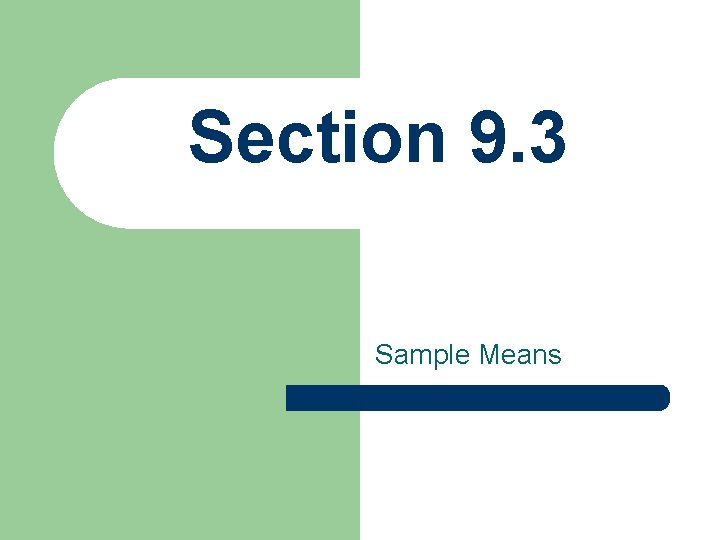Section 9. 3 Sample Means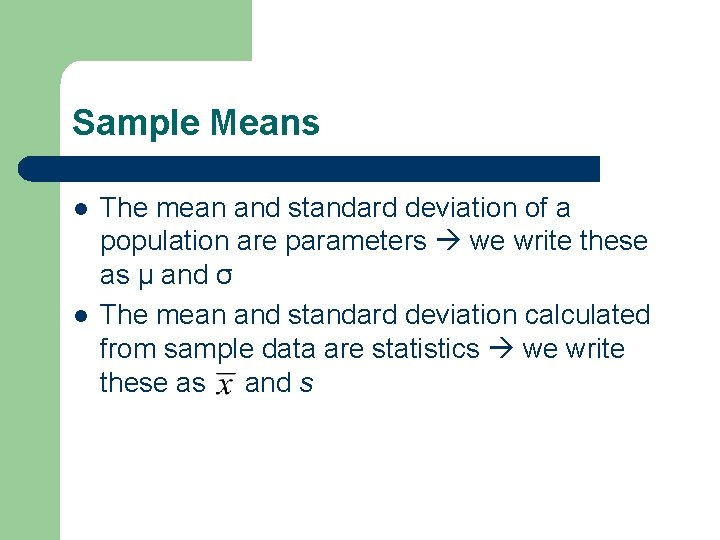Sample Means l l The mean and standard deviation of a population are parameters we write these as µ and σ The mean and standard deviation calculated from sample data are statistics we write these as and s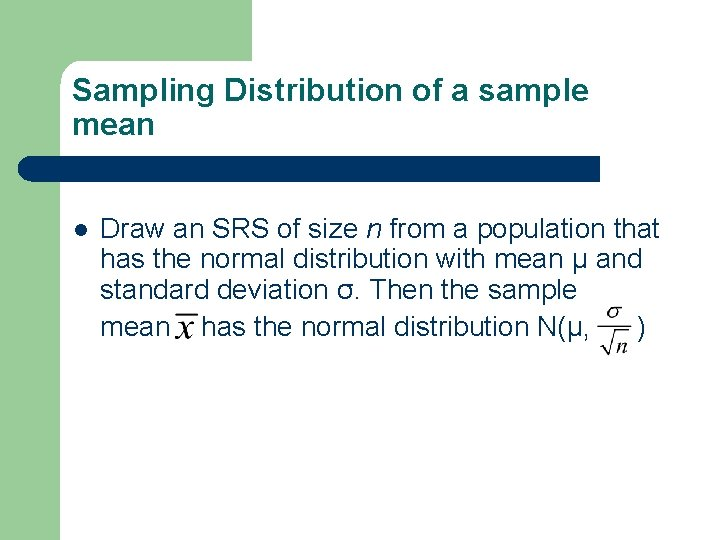Sampling Distribution of a sample mean l Draw an SRS of size n from a population that has the normal distribution with mean µ and standard deviation σ. Then the sample mean has the normal distribution N(µ, )THE CENTRAL LIMIT THEOREM l Central Limit Theorem states that for large n the sampling distribution of is approximately normal for any population with finite standard deviation σ. The mean and standard deviation of the normal distribution are the mean µ and standard deviation. Like before (with proportions), we want our population to be at least ten times as big as the sample to use this recipe for standard deviationLaw of Large Numbers l Law of Large Numbers states that the actually observed mean outcome of a large number of observations must approach the mean µ of the population as the number of observations increases.Let’s Try Some Multiple Choice NUMBER ONE l When a sample is randomly selected from a population, what is most likely to occur? – – A. The sample distribution will be identical to the population distribution. B. The sample distribution will be quite similar to the population distribution at first, but will become less similar as the sample gets larger. C. The sample distribution could be quite different from the population distribution at first, but will become more similar as the sample gets larger. D. The sample distribution will not be at all similar to the population distribution.AND THE ANSWER IS. . . l Correct Answer: C — The sample distribution could be quite different from the population distribution at first, but will become more similar as the sample gets larger. Explanation: Small samples are often quite different from the populations they are from, but as the sample becomes larger the sample tends to resemble the population distribution more and more. This is true in terms of the overall shape of the distribution, as well as the sample mean compared with the population mean.Let’s Try Some Multiple Choice NUMBER TWO l When samples are randomly selected from a population, what is most likely to occur? – – A. The sampling distribution will be identical to the population distribution. B. The sampling distribution will be quite similar to the population distribution at first, but will become more normal as the samples gets larger. C. The sampling distribution could be quite different from the population distribution at first, but will become more similar as the samples gets larger. D. The sampling distribution will not be at all similar to the population distribution.AND THE ANSWER IS. . . l l Correct Answer: B — The sampling distribution will be quite similar to the population distribution at first, but will become more normal as the samples get larger. Explanation: Don’t forget, the sampling distribution is different than a sample distribution. The Central Limit Theorem reminds us that the sampling distribution will be approximately normal for large sample sizes regardless of the shape of the population distribution. This is what makes the Central Limit Theorem so important!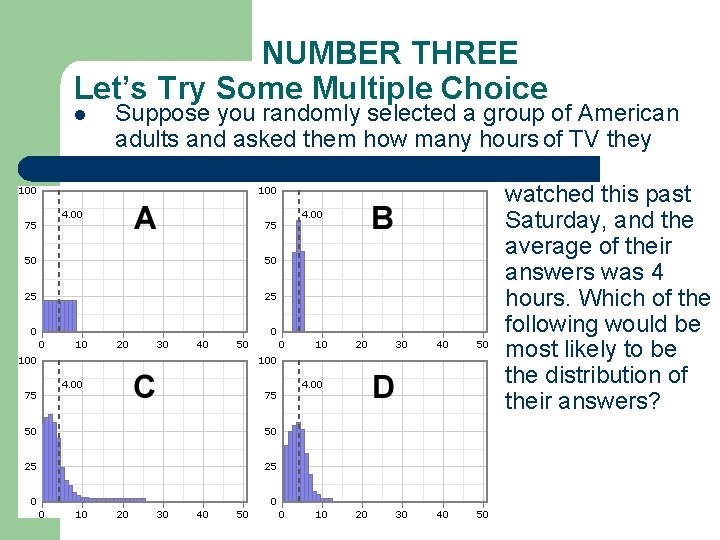NUMBER THREE Let’s Try Some Multiple Choice l Suppose you randomly selected a group of American adults and asked them how many hours of TV they watched this past Saturday, and the average of their answers was 4 hours. Which of the following would be most likely to be the distribution of their answers?AND THE ANSWER IS. . . l Correct Answer: D — Distribution D Explanation: Distribution D shows most responses between 0 and 5 hours, with a short tail on the right. Distribution A is not likely because it is extremely unlikely that the number of people who watch 8 hours of TV in a day is the same as the number of people who watch 1 hour of TV. Distribution B is too tightly bunched; surely not everyone you sample would have watched 3, 4, or 5 hours or TV. Finally, distribution C is impossible because it appears that someone in the sample responded that he or she watched 25 hours of TV on Saturday.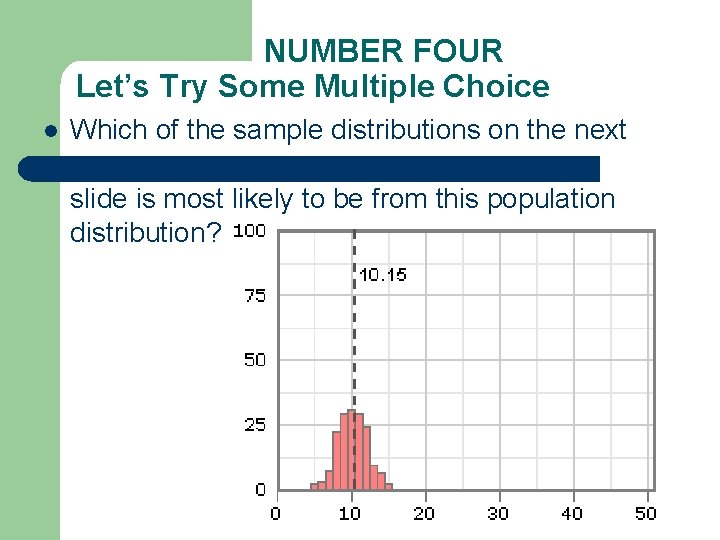NUMBER FOUR Let’s Try Some Multiple Choice l Which of the sample distributions on the next slide is most likely to be from this population distribution?Choices for Number Four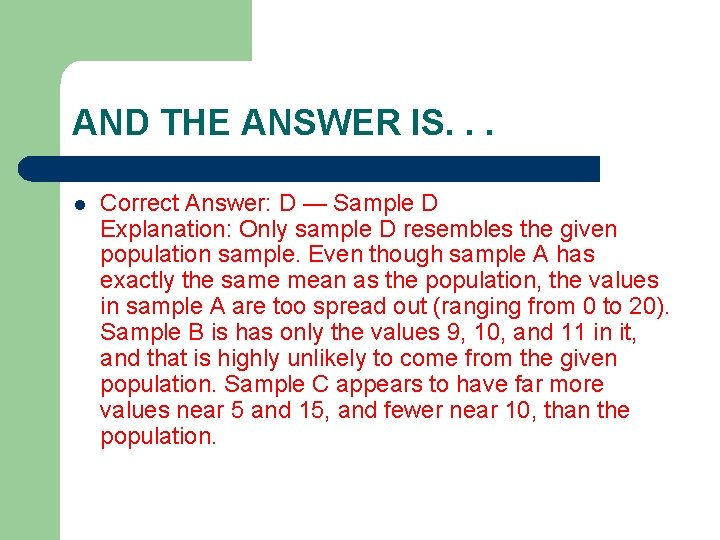AND THE ANSWER IS. . . l Correct Answer: D — Sample D Explanation: Only sample D resembles the given population sample. Even though sample A has exactly the same mean as the population, the values in sample A are too spread out (ranging from 0 to 20). Sample B is has only the values 9, 10, and 11 in it, and that is highly unlikely to come from the given population. Sample C appears to have far more values near 5 and 15, and fewer near 10, than the population.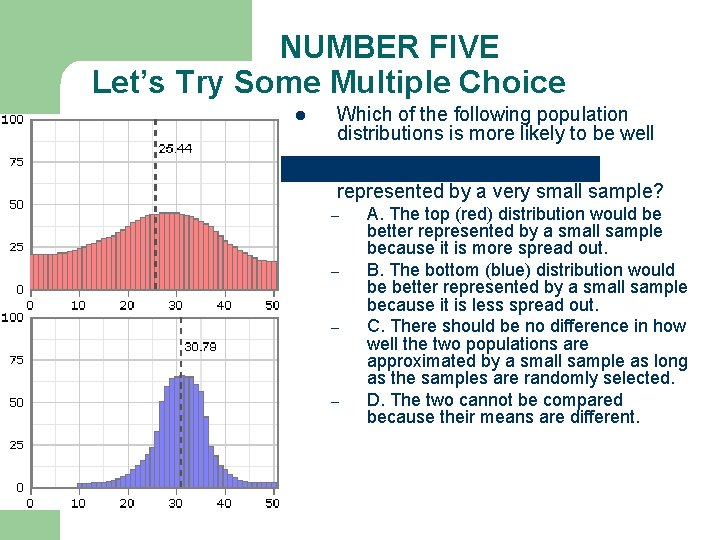NUMBER FIVE Let’s Try Some Multiple Choice l Which of the following population distributions is more likely to be well represented by a very small sample? – – A. The top (red) distribution would be better represented by a small sample because it is more spread out. B. The bottom (blue) distribution would be better represented by a small sample because it is less spread out. C. There should be no difference in how well the two populations are approximated by a small sample as long as the samples are randomly selected. D. The two cannot be compared because their means are different.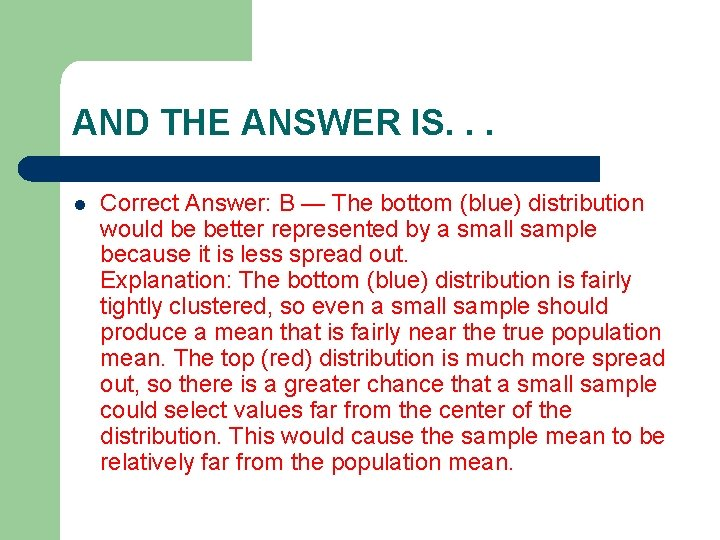AND THE ANSWER IS. . . l Correct Answer: B — The bottom (blue) distribution would be better represented by a small sample because it is less spread out. Explanation: The bottom (blue) distribution is fairly tightly clustered, so even a small sample should produce a mean that is fairly near the true population mean. The top (red) distribution is much more spread out, so there is a greater chance that a small sample could select values far from the center of the distribution. This would cause the sample mean to be relatively far from the population mean.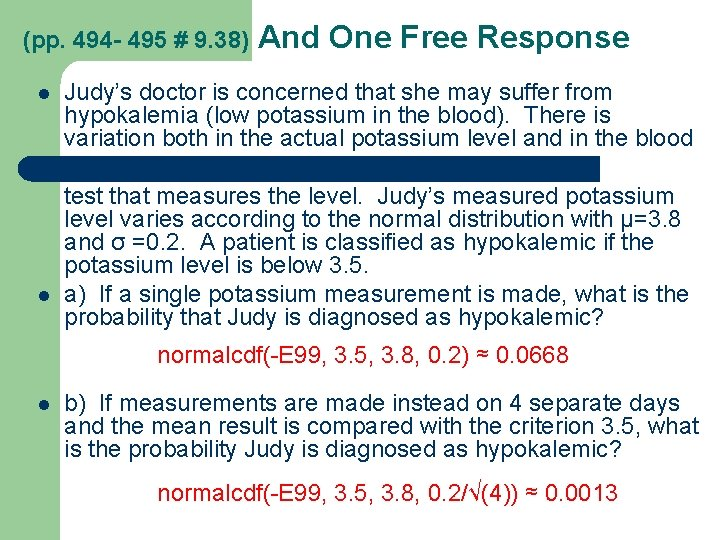(pp. 494 - 495 # 9. 38) l l And One Free Response Judy’s doctor is concerned that she may suffer from hypokalemia (low potassium in the blood). There is variation both in the actual potassium level and in the blood test that measures the level. Judy’s measured potassium level varies according to the normal distribution with μ=3. 8 and σ =0. 2. A patient is classified as hypokalemic if the potassium level is below 3. 5. a) If a single potassium measurement is made, what is the probability that Judy is diagnosed as hypokalemic? normalcdf(-E 99, 3. 5, 3. 8, 0. 2) ≈ 0. 0668 l b) If measurements are made instead on 4 separate days and the mean result is compared with the criterion 3. 5, what is the probability Judy is diagnosed as hypokalemic? normalcdf(-E 99, 3. 5, 3. 8, 0. 2/√(4)) ≈ 0. 0013